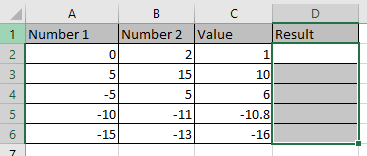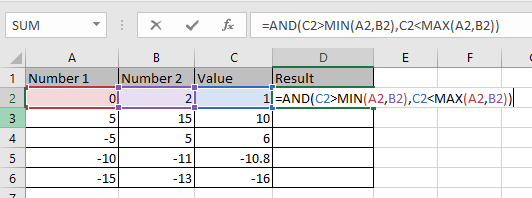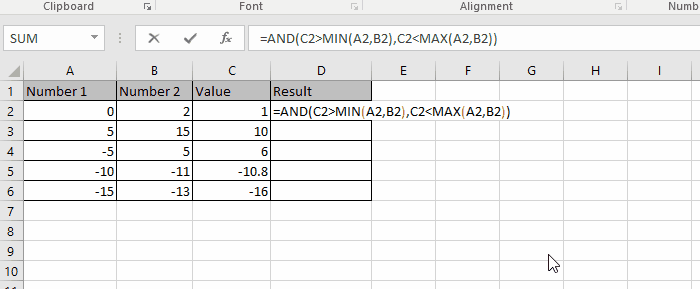# How to Check If value is between the two numbers in Excel

In this article, we will learn how to check if the value is between the two numbers in Excel.

We have 2 numbers and a value to check that it lies between the given two numbers or not. For this article, we need to satisfy two conditions using AND logic operator. AND logic operator checks multiple conditions and returns TRUE only if all the condition stands TRUE or FALSE otherwise.

The two conditions which needed to check are The value must be smaller than the larger of the number and Greater than the smaller of the numbers. For this we will be using the MIN & MAX function in excel

Syntax:

=AND ( logic_test1, logic_test2,...)

Let’s learn this through an example shown below.

Here we have the two numbers given in Column A and Column B and the value to check in Column C.Use the formula

=AND(C2>MIN(A2,B2),C2<MAX(A2,B2))

Explanation:

We just need to check 2 condition

• The value is greater than the smaller of the two numbers.
• The value is smaller than the larger of the two numbers.

Here MAX & MIN function returns the maximum and minimum of the two numbers. AND functions test both conditions and returns “True” only if both statements stands True else False.Press Enter and copy the formula in other cells using Ctrl + D shortcut.As you can see the formula returns True if the value lies between the two numbers else it returns False.

Hope you learned how to check the value lies between the two numbers in Excel. Explore more articles here on Excel Logic_test functions here. Please feel free to state your query or feedback in the comment section below. We will help you.

Related Articles

How to Ignore zero in the Average of numbers in Excel

How to Get Random numbers between two numbers in Excel

How to Calculate a ratio of two numbers in Excel

How to Generate Random Phone Numbers in Excel

Popular Articles

50 Excel Shortcut to Increase Your Productivity

Edit a dropdown list

If with conditional formatting

If with wildcards

Vlookup by date

Terms and Conditions of use

The applications/code on this site are distributed as is and without warranties or liability. In no event shall the owner of the copyrights, or the authors of the applications/code be liable for any loss of profit, any problems or any damage resulting from the use or evaluation of the applications/code.